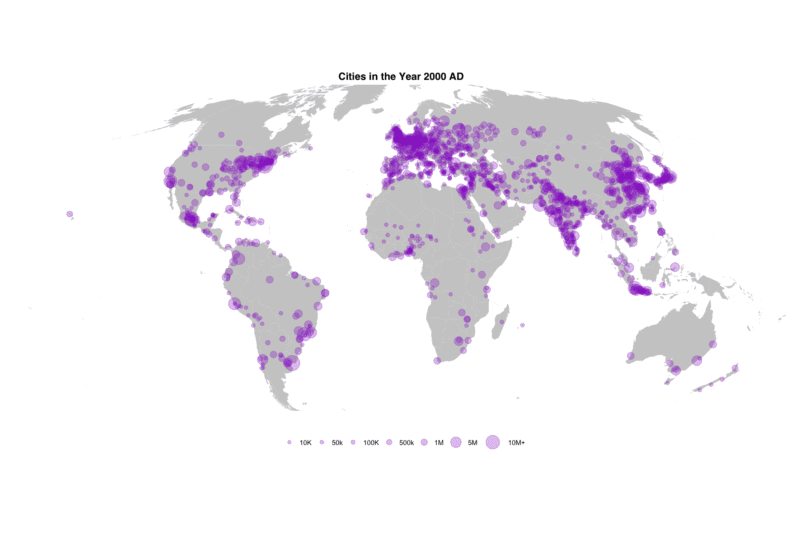# Mapping 5,000 Years of City GrowthI recently stumbled upon a great dataset. It’s the first to provide comprehensive data for world city sizes as far back as 3700BC. The authors (Meredith Reba, Femke Reitsma & Karen Seto) write:
How were cities distributed globally in the past? How many people lived in these cities? How did cities influence their local and regional environments? In order to understand the current era of urbanization, we must understand long-term historical urbanization trends and patterns. However, to date there is no comprehensive record of spatially explicit, historic, city-level population data at the global scale. Here, we developed the first spatially explicit dataset of urban settlements from 3700 BC to AD 2000, by digitizing, transcribing, and geocoding historical, archaeological, and census-based urban population data previously published in tabular form by Chandler and Modelski.
These kinds of data are crying out to be mapped so that’s what I did…## Creating the Maps

I was keen to see how easy it was to produce an animated map with R and the ggplot2 package from these data. It turned out to be a slightly bigger job than I thought – partly because the cleaned data doesn’t have all the population estimates updating for every city each year so there’s a bit of a messy loop at the start of the code to get that bit working, and partly because there a LOADS of different parameters to tweak on ggplot to get the maps looking the way I like. The script below will generate a series of PNGs that can be strung together into an animation using a graphics package. To learn how to convert these into an animation see this excellent tutorial by Lena Groeger. I’m a big fan of what ggplot2 can do now and I hope, at the very least, the chunks of ggplot2 code below will provide a useful template for similar mapping projects.

### R Code

``````library("ggplot2")
library("ggthemes")
library("rworldmap")
library("classInt")
library("gridExtra")
library("grid")
library("cowplot")``````

load data – this is the processed file from http://www.nature.com/articles/sdata201634 I offer no guarantees about whether I ran the script correctly etc! I’d encourage you to take the data direct from the authors.

``````#City data
#for some reason the coords were loaded as factors so I've created two new numeric fields here
cities\$X<-as.numeric(as.vector(cities\$Longitude))
cities\$Y<-as.numeric(as.vector(cities\$Latitude))
#World map base from rworldmap
world <- fortify(getMap(resolution="low"))``````

Take a look at the data

``head(cities)``

Create the base map

``base<- ggplot() + geom_map(data=world, map=world, aes(x=long,y=lat, map_id=id, group=group),fill="#CCCCCC") +theme_map()``

Now plot the cities on top – with circles scaled by city size. Here I am using the mollweide projection. This script loops through the data and checks for updates from one year to the next. It then plots the cities as proportional circles for each year and saves out an image for each 100 years of data. If you are just interested in the maps themselves and have your own point data then most of the below can be ignored.
Set the breaks for the size of the circles

``size_breaks<-c(10000,50000,100000,500000,1000000,5000000,10000000)``
``````#Here I'm looping through the data each year to select the years required and comparing/ updating the values for each city.
count<-1
for (i in unique(cities\$year))
{
if (count==1)
{
Data<-cities[which(cities\$year==i),]
}else{
New_Data<-cities[which(cities\$year==i),]
#replace old rows
Data2<-merge(Data, New_Data, by.x="X", by.y="X", all=T)
New_Vals<-Data2[!is.na(Data2\$pop.y),c("City.y","X","Y.y","pop.y")]
names(New_Vals)<-c("City","X","Y","pop")
Old_Vals<-Data2[is.na(Data2\$pop.y),c("City.x","X","Y.x","pop.x")]
names(Old_Vals)<-c("City","X","Y","pop")
Data<-rbind(New_Vals,Old_Vals)
Data<- aggregate(Data\$pop, by=list(Data\$City,Data\$X, Data\$Y), FUN="mean")
names(Data)<-c("City","X","Y","pop")
}
#This statement sorts out the BC/ AC in the title that we'll use for the plot.
if(i<0)
{
title <- paste0("Cities in the Year ",sqrt(i^2)," BC")
}else{
title <- paste0("Cities in the Year ",i," AD")
}
#There's lots going on here with the myriad of different ggplot2 parameters. I've broken each chunk up line by line to help make it a little clearer.
Map<-base+
geom_point(data=Data, aes(x=X, y=Y, size=pop), colour="#9933CC",alpha=0.3, pch=20)+
scale_size(breaks=size_breaks,range=c(2,14), limits=c(min(cities\$pop), max(cities\$pop)),labels=c("10K","50k","100K","500k","1M","5M","10M+"))+
coord_map("mollweide", ylim=c(-55,80),xlim=c(-180,180))+
theme(legend.position="bottom",legend.direction="horizontal",legend.justification="center",legend.title=element_blank(),plot.title = element_text(hjust = 0.5,face='bold',family = "Helvetica"))+ggtitle(title)+guides(size=guide_legend(nrow=1))
#I only want to plot map every 100 years
if(i%%100==0)
{
png(paste0("outputs/",i,"_moll.png"), width=30,height=20, units="cm", res=150)
print(Map)
dev.off()
}
count<-count+1
}``````

Just to make things a little more interesting I wanted to try a plot of two hemispheres (covering most, but not all) of the globe. I’ve also added the key in between them. This depends on the `plot_grid()` functionality as well as a few extra steps you’ll spot that we didn’t need above.

``````count<-1
for (i in unique(cities\$year))
{
if (count==1)
{
Data<-cities[which(cities\$year==i),]
}else{
New_Data<-cities[which(cities\$year==i),]
#replace old rows
Data2<-merge(Data, New_Data, by.x="X", by.y="X", all=T)
New_Vals<-Data2[!is.na(Data2\$pop.y),c("City.y","X","Y.y","pop.y")]
names(New_Vals)<-c("City","X","Y","pop")
Old_Vals<-Data2[is.na(Data2\$pop.y),c("City.x","X","Y.x","pop.x")]
names(Old_Vals)<-c("City","X","Y","pop")
Data<-rbind(New_Vals,Old_Vals)
Data<- aggregate(Data\$pop, by=list(Data\$City,Data\$X, Data\$Y), FUN="mean")
names(Data)<-c("City","X","Y","pop")
}
#Create a map for each hemisphere - to help with the positioning I needed to use the `plot.margin` options in the `theme()` settings.
map1<-base+geom_point(data=Data, aes(x=X, y=Y, size=pop), colour="#9933CC",alpha=0.3, pch=20)+scale_size(breaks=size_breaks,range=c(2,16), limits=c(min(cities\$pop), max(cities\$pop)))+coord_map("ortho", orientation = c(10, 60, 0),ylim=c(-55,70))+ theme(legend.position="none",plot.margin = unit(c(0.5,0.5,-0.1,0.5), "cm"))
map2<- base+geom_point(data=Data, aes(x=X, y=Y, size=pop), colour="#9933CC",alpha=0.3, pch=20)+scale_size(breaks=size_breaks,range=c(2,16),limits=c(min(cities\$pop), max(cities\$pop)))+coord_map("ortho", orientation = c(10, -90, 0),ylim=c(-55,70))+ theme(legend.position="none", plot.margin = unit(c(0.5,0.5,0,0.5), "cm"))
#create dummy plot that we will use the legend from - basically I just want to create something that has a legend (they've been switched off for the maps above)
dummy<-ggplot()+geom_point(aes(1:7,1,size=size_breaks),colour="#9933CC", alpha=0.3, pch=20)+scale_size(breaks=size_breaks,range=c(2,16),labels=c("10K","50k","100K","500k","1M","5M","10M+"),limits=c(min(cities\$pop), max(cities\$pop)))+theme(legend.position="bottom",legend.direction="vertical",legend.title=element_blank())+guides(size=guide_legend(nrow=1,byrow=TRUE))
Legend <- get_legend(dummy)
#This statement sorts out the BC/ AC in the title.
if(i<0)
{
title <- ggdraw() + draw_label(paste0("Cities in the Year ",sqrt(i^2)," BC"), fontface='bold',fontfamily = "Helvetica")
}else{
title <- ggdraw() + draw_label(paste0("Cities in the Year ",i," AD"), fontface='bold',fontfamily = "Helvetica")
}
#I only want to plot map every 100 years
if(i%%100==0)
{
png(paste0("outputs/",i,".png"), width=20,height=30, units="cm", res=150)
#This is where the elements of the plot are combined
print(plot_grid(title,map1, Legend,map2, ncol=1,rel_heights = c(.1,1, .1,1)))
dev.off()
}
count<-count+1
}``````James Cheshire